SNVS974B April   2013  – October 2015

PRODUCTION DATA.

1. Features
2. Applications
3. Description
4. Revision History
5. Pin Configuration and Functions
6. Specifications
7. Detailed Description
1. 7.1 Overview
2. 7.2 Functional Block Diagram
3. 7.3 Feature Description
1. 7.3.1 Operation
2. 7.3.2 Test Features
3. 7.3.3 Fault Flags/Protection Features
4. 7.3.4 Initialization Timing
4. 7.4 Device Functional Modes
1. 7.4.1 LED Current Ramping
2. 7.4.2 PWM Operation
5. 7.5 Programming
1. 7.5.1 I2C-Compatible Interface
6. 7.6 Register Maps
8. Application and Implementation
1. 8.1 Application Information
2. 8.2 Typical Application
1. 8.2.1 Design Requirements
2. 8.2.2 Detailed Design Procedure
3. 8.2.3 Application Curves
3. 8.3 Initialization Setup
9. Power Supply Recommendations
10. 10Layout
1. 10.1 Layout Guidelines
2. 10.2 Layout Example
11. 11Device and Documentation Support
1. 11.1 Device Support
2. 11.2 Documentation Support
3. 11.3 Community Resources
5. 11.5 Electrostatic Discharge Caution
6. 11.6 Glossary
12. 12Mechanical, Packaging, and Orderable Information

• YFQ|12

## 6 Specifications

### 6.1 Absolute Maximum Ratings

over operating free-air temperature range (unless otherwise noted)(1)(2)
MIN MAX UNIT
IN, HWEN, PWM, SCL, SDA, INTN, SEL to GND –0.3 6 V
SW, OVP, ILED1, ILED2 to GND –0.3 45 V
T(J-MAX) Continuous power dissipation(3) Internally limited
Maximum junction temperature 150
Vapor phase (60 sec.)
215 °C
Infrared (15 sec.)
220 °C
Storage temperature, Tstg −45 150 °C
(1) Stresses beyond those listed under Absolute Maximum Ratings may cause permanent damage to the device. These are stress ratings only, which do not imply functional operation of the device at these or any other conditions beyond those indicated under Recommended Operating Conditions. Exposure to absolute-maximum-rated conditions for extended periods may affect device reliability.
(2) All voltages are with respect to the potential at the GND pin.
(3) Internal thermal shutdown circuitry protects the device from permanent damage. Thermal shutdown engages at TJ = 140°C (typical) and disengages at TJ = 125°C (typical).
(4) For detailed soldering specifications and information, refer to Texas Instruments Application Note 1112: DSBGA Wafer Level Chip Scale Package (SNVA009).

### 6.2 ESD Ratings

VALUE UNIT
V(ESD) Electrostatic discharge Human-body model (HBM), per ANSI/ESDA/JEDEC JS-001(1) ±2000 V
Charged-device model (CDM), per JEDEC specification JESD22-C101(2) ±500
(1) JEDEC document JEP155 states that 500-V HBM allows safe manufacturing with a standard ESD control process.
(2) JEDEC document JEP157 states that 250-V CDM allows safe manufacturing with a standard ESD control process.

### 6.3 Recommended Operating Conditions

over operating free-air temperature range (unless otherwise noted)
MIN NOM MAX UNIT
VIN Input voltage 2.3 5.5 V
TA Operating ambient temperature −40 85 °C

### 6.4 Thermal Information

THERMAL METRIC(1) LM3630A UNIT
YFQ (DBSGA)
12 PINS
RθJA Junction-to-ambient thermal resistance 78.1 °C/W

### 6.5 Electrical Characteristics

Typical limits are for TA = 25°C; minimum and maximum limits apply over the full operating ambient temperature range (−40°C ≤ TA ≤ 85°C); VIN = 3.6 V, unless otherwise specified.(1)
PARAMETER TEST CONDITION MIN TYP MAX UNIT
ILED1, ILED2 Output current regulation 2.5 V ≤ VIN ≤ 5.5 V, full-scale current = 20 mA 19 20 21 mA
IMATCH ILED1 to ILED2 current matching (2) 2.5 V ≤ VIN ≤ 5.5 V, ILED = 10 mA, TA = 25°C ILED1 on A
ILED2 on B
–1% 0.5% 1%
2.5 V ≤ VIN ≤ 5.5 V, ILED = 10 mA, 0°C ≤ TA ≤ 70°C –2.5% 0.5% 2.5%
VREG_CS Regulated current sink headroom voltage ILED = 5 mA 250 mV
VHR Current sink minimum headroom voltage ILED = 95% of nominal, ILED = 20 mA 160 240
RDSON NMOS switch on resistance ISW = 100 mA 0.25 Ω
ICL NMOS switch current limit 2.5 V ≤ VIN ≤ 5.5 V 480 600 720 mA
640 800 960
800 1000 1200
960 1200 1440
VOVP Output overvoltage protection ON threshold, 2.3 V ≤ VIN ≤ 5.5 V 24-V option 23 24 25 V
ON threshold, 2.3 V ≤ VIN ≤ 5.5 V 40-V option 39 41 44
Hysteresis 1
ƒSW Switching frequency 2.5 V ≤ VIN ≤ 5.5 V 560-kHz shift = 1 538 560 582 kHz
500-kHz shift = 0 481 500 518
1.12-MHz shift = 1 1077 1120 1163
1-MHz shift = 0 962 1000 1038
DMAX Maximum duty cycle 94%
IQ Quiescent current into device, not switching VIN = 3.6 V ILED1 = ILED2 =
20 mA, feedback disabled.
350 µA
ISHDN Shutdown current 2.3 V ≤ VIN ≤ 5.5 V HWEN = VIN, I2C shutdown 1 4 µA
HWEN = GND 1 4
ILED_MIN Minimum LED current in ILED1 or ILED2 Full-scale current = 20 mA, BRT = 0x01, Exponential mapping mode 13
TSD Thermal shutdown 140 °C
Hysteresis 15
tWAIT Initialization timing Time period to wait from the assertion of HWEN or after software reset, before an I2C transaction will be ACK'ed. During this time period an I2C transaction will be NAK'ed 1 ms
LOGIC INPUTS (PWM, HWEN, SEL, SCL, SDA)
VIL Input logic low 2.3 V ≤ VIN ≤ 5.5 V 0 0.4 V
VIH Input logic high 2.3 V ≤ VIN ≤ 5.5 V 1.2 VIN
VOL Output logic low (SDA, INTN) 2.3 V ≤ VIN ≤ 5.5 V 400 mV
ƒPWM PWM input frequency 2.3 V ≤ VIN ≤ 5.5 V 10 80 kHz
CIN Input capacitance SDA 4.5 pF
SCL 5
(1) Minimum and maximum limits are specified by design, test, or statistical analysis. Typical numbers are not ensured, but do represent the most likely norm. Unless otherwise specified, conditions for typical specifications are: VIN = 3.6 V and TA = 25°C.
(2) LED current sink matching between LED1 and LED2 is given by taking the difference between ILED1 and ILED2 and dividing by the sum of ILED1 and ILED2. The formula is (ILED1 − ILED2)/(ILED1 + ILED2) at ILED = 10 mA. ILED1 is driven by Bank A and ILED2 is driven by Bank B.

### 6.6 I2C-Compatible Timing Requirements (SCL, SDA)

See(1).
MIN NOM MAX UNIT
t1 SCL (clock period) 2.5 µs
t2 Data in setup time to SCL high 100 ns
t3 Data in setup time to SCL low 0
t4 SDA low setup time to SCL low (start) 100
t5 SDA high hold time to SCL high (stop) 100
(1) SCL and SDA must be glitch-free in order for proper brightness to be realized.

### 6.7 Typical Characteristics

TA = 25°C, ILED full-scale = 20 mA, unless specified otherwise.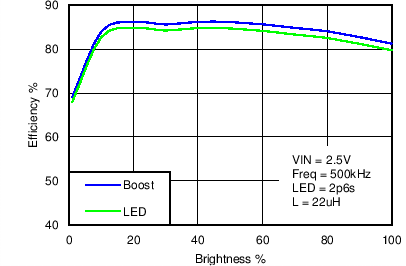VIN = 2.5 V 2p6s L = 22 µH Frequency = 500 kHz
Figure 1. Boost and LED Efficiency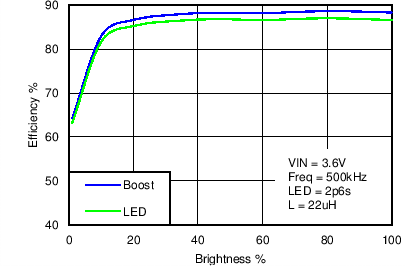VIN = 3.6 V 2p6s L = 22 µH Frequency = 500 kHz
Figure 3. Boost and LED Efficiency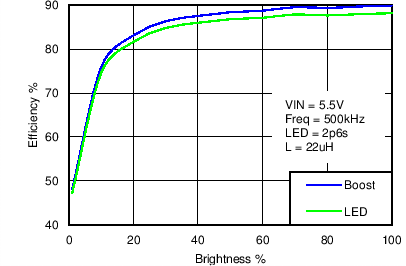VIN = 5.5 V 2p6s L = 22 µH Frequency = 500 kHz
Figure 5. Boost and LED Efficiency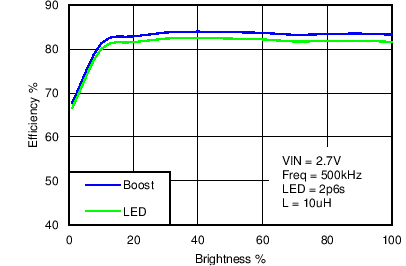VIN = 2.7 V 2p6s L = 10 µH Frequency = 500 kHz
Figure 7. Boost and LED Efficiency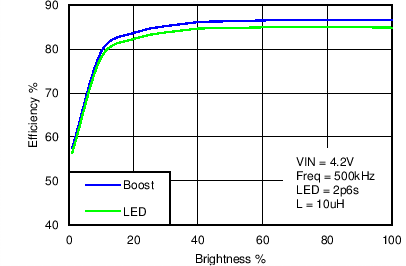VIN = 4.2 V 2p6s L = 10 µH Frequency = 500 kHz
Figure 9. Boost and LED Efficiency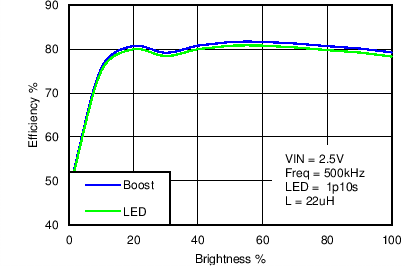VIN = 2.5 V 1p10s L = 22 µH Frequency = 500 kHz
Figure 11. Boost and LED Efficiency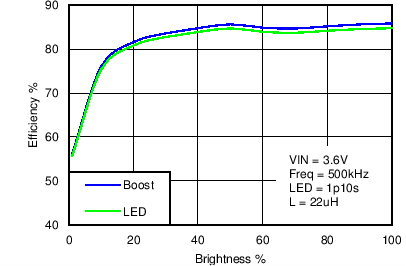VIN = 3.6 V 1p10s L = 22 µH Frequency = 500 kHz
Figure 13. Boost and LED Efficiency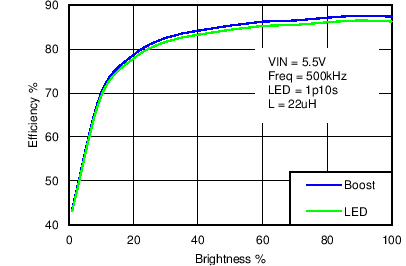VIN = 5.5 V 1p10s L = 22 µH Frequency = 500 kHz
Figure 15. Boost and LED Efficiency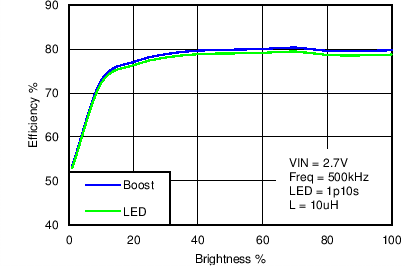VIN = 2.7 V 1p10s L = 10 µH Frequency = 500 kHz
Figure 17. Boost and LED Efficiency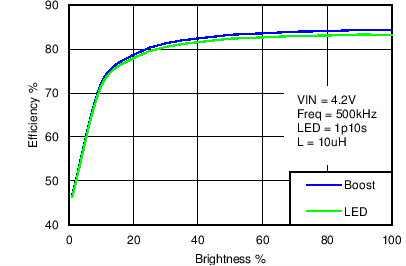VIN = 4.2 V 1p10s L = 10 µH Frequency = 500 kHz
Figure 19. Boost and LED Efficiency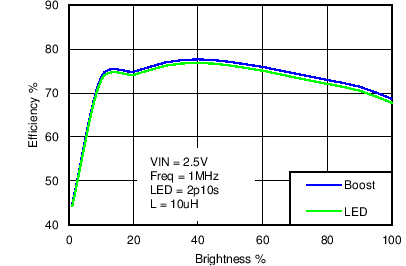VIN = 2.5 V 2p10s L = 10 µH Frequency = 1 MHz
Figure 21. Boost and LED Efficiency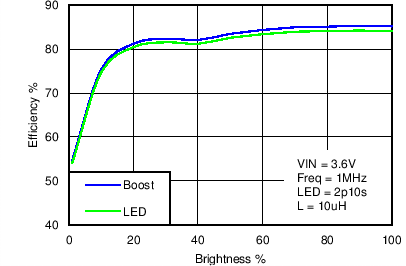VIN = 3.6 V 2p10s L = 10 µH Frequency = 1 MHz
Figure 23. Boost and LED Efficiency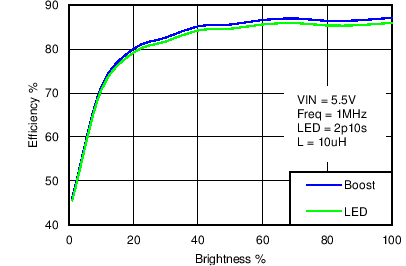VIN = 5.5 V 2p10s L = 10 µH Frequency = 1 MHz
Figure 25. Boost and LED Efficiency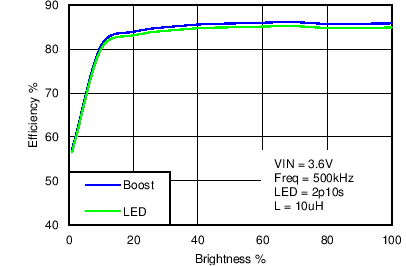VIN = 3.6 V 2p10s L = 10 µH Frequency = 500 kHz
Figure 27. Boost and LED Efficiency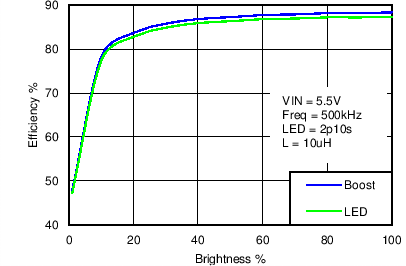VIN = 5.5 V 2p10s L = 10 µH Frequency = 500 kHz
Figure 29. Boost and LED Efficiency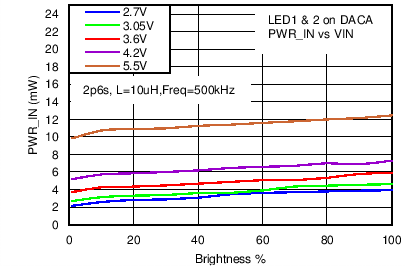ILED Full Scale = 28.5 mA LED1 and 2 on DACA Frequency = 500 kHz 2p6s L = 10 µH
Figure 31. PWR_IN Across VIN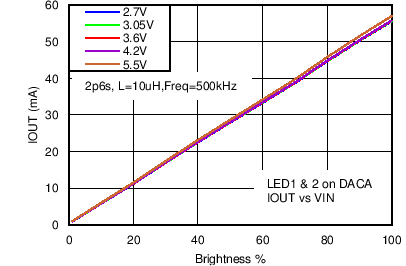ILED Full Scale = 28.5 mA LED1 and 2 on DACA Frequency = 500 kHz 2p6s L = 10 µH
Figure 33. IOUT Across VIN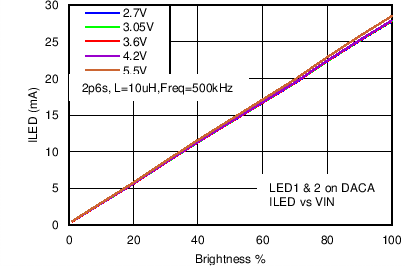ILED Full Scale = 28.5 mA LED1 and 2 on DACA Frequency = 500 kHz 2p6s L = 10 µH
Figure 35. ILED Across VIN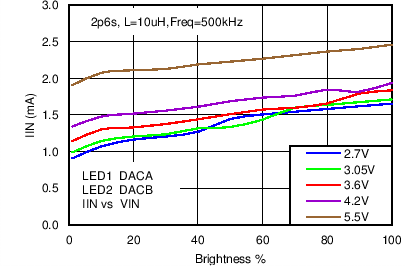ILED Full Scale = 28.5 mA LED1 on DACA 2p6s Frequency = 500 kHz LED2 on DACB L = 10 µH
Figure 37. IIN Across VIN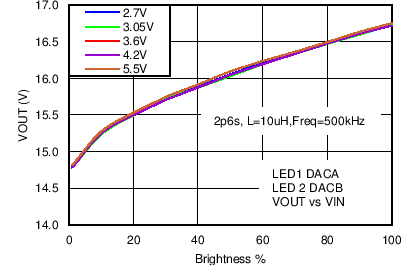ILED Full Scale = 28.5 mA LED1 on DACA 2p6s Frequency = 500 kHz LED2 on DACB L = 10 µH
Figure 39. VOUT Across VIN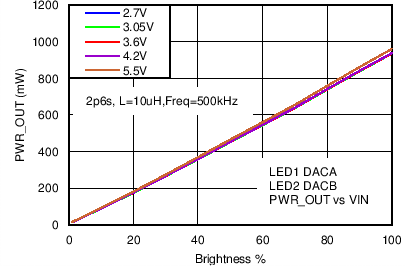ILED Full Scale = 28.5 mA LED1 on DACA 2p6s Frequency = 500 kHz LED2 on DACB L = 10 µH
Figure 41. PWR_OUT Across VIN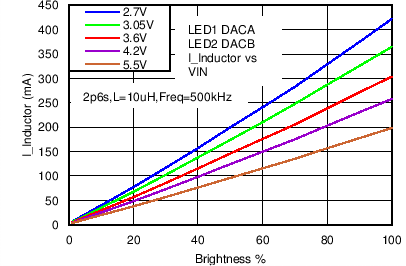ILED Full Scale = 28.5 mA LED1 on DACA 2p6s Frequency = 500 kHz LED2 on DACB L = 10 µH
Figure 43. I_INDUCTOR Across VIN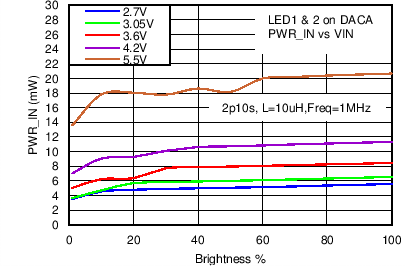ILED Full Scale = 28.5 mA LED1 and LED2 on DACA Frequency = 1 MHz 2p10s L = 10 µH
Figure 45. PWR_IN Across VIN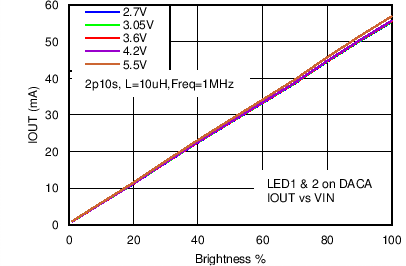ILED Full Scale = 28.5 mA LED1 and LED2 on DACA Frequency = 1 MHz 2p10s L = 10 µH
Figure 47. IOUT Across VIN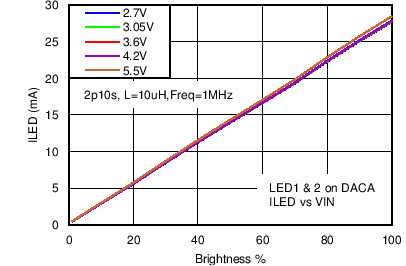ILED Full Scale = 28.5 mA LED1 and LED2 on DACA Frequency = 1 MHz 2p10s L = 10 µH
Figure 49. ILED Across VIN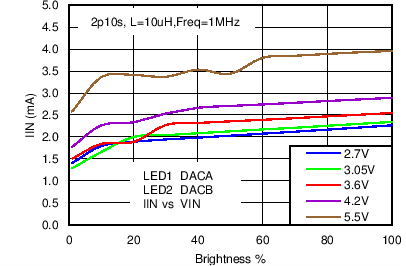ILED Full Scale = 28.5 mA LED1 on DACA 2p10s Frequency = 1 MHz LED2 on DACB L = 10 µH
Figure 51. IIN Across VIN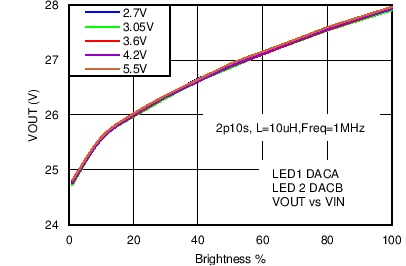ILED Full Scale = 28.5 mA LED1 on DACA 2p10s Frequency = 1 MHz LED2 on DACB L = 10 µH
Figure 53. VOUT Across VIN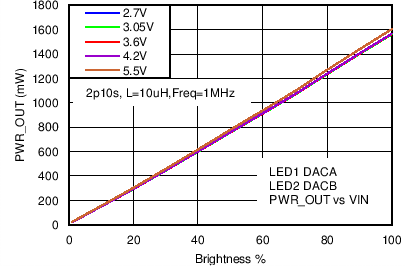ILED Full Scale = 28.5 mA LED1 on DACA 2p10s Frequency = 1 MHz LED2 on DACB L = 10 µH
Figure 55. PWR_OUT Across VIN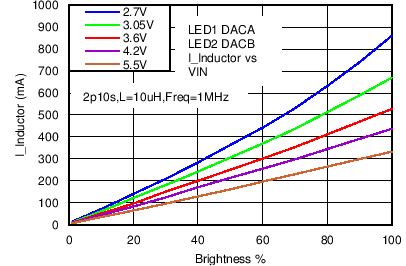ILED Full Scale = 28.5 mA LED1 on DACA 2p10s Frequency = 1 MHz LED2 on DACB L = 10 µH
Figure 57. I_INDUCTOR Across VIN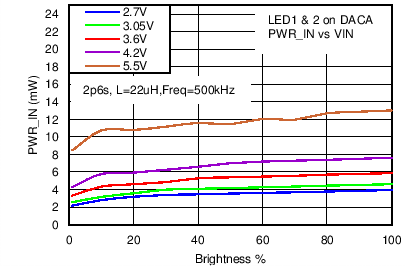ILED Full Scale = 28.5 mA LED1 and LED2 on DACA Frequency = 500 kHz 2p6s L = 22 µH
Figure 59. PWR_IIN Across VIN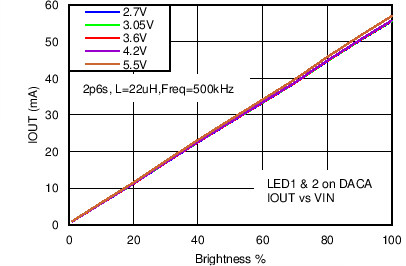ILED Full Scale = 28.5 mA LED1 and LED2 on DACA Frequency = 500 kHz 2p6s L = 22 µH
Figure 61. IOUT Across VINILED Full Scale = 28.5 mA LED1 and LED2 on DACA Frequency = 500 kHz 2p6s L = 22 µH
Figure 63. ILED Across VIN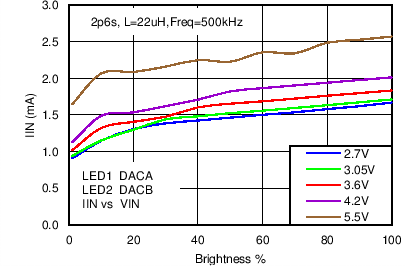ILED Full Scale = 28.5 mA LED1 on DACA 2p6s Frequency = 500 kHz LED2 on DACB L = 22 µH
Figure 65. IIN Across VIN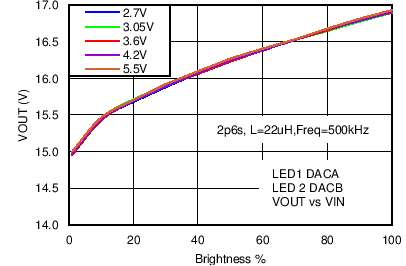ILED Full Scale = 28.5 mA LED1 on DACA 2p6s Frequency = 500 kHz LED2 on DACB L = 22 µH
Figure 67. VOUT Across VIN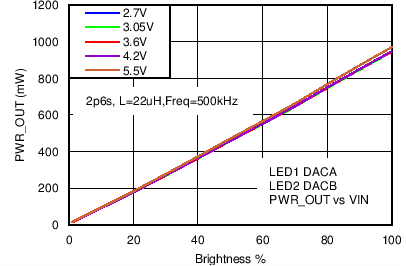ILED Full Scale = 28.5 mA LED1 on DACA 2p6s Frequency = 500 kHz LED2 on DACB L = 22 µH
Figure 69. PWR_OUT Across VIN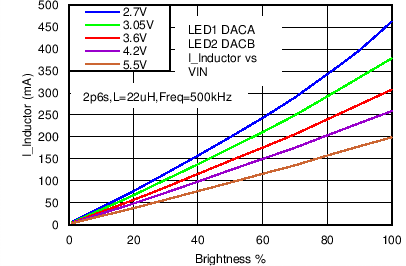ILED Full Scale = 28.5 mA LED1 on DACA 2p6s Frequency = 500 kHz LED2 on DACB L = 22 µH
Figure 71. I_INDUCTOR Across VIN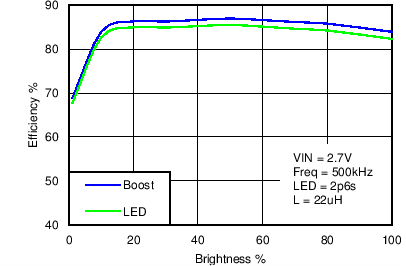VIN = 2.7 V 2p6s L = 22 µH Frequency = 500 kHz
Figure 2. Boost and LED Efficiency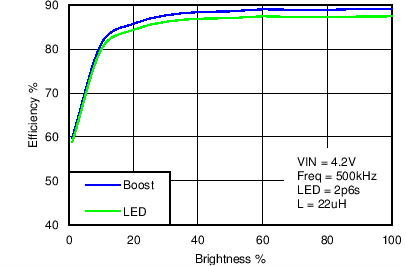VIN = 4.2 V 2p6s L = 22 µH Frequency = 500 kHz
Figure 4. Boost And LED Efficiency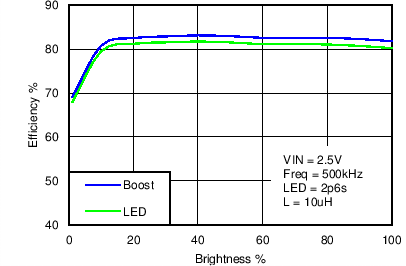VIN = 2.5 V 2p6s L = 10 µH Frequency = 500 kHz
Figure 6. Boost and LED Efficiency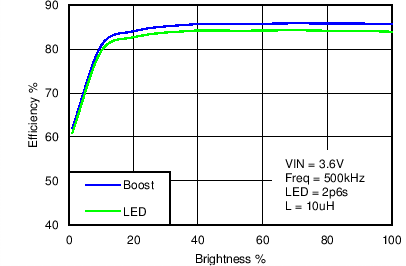VIN = 3.6 V 2p6s L = 10 µH Frequency = 500 kHz
Figure 8. Boost and LED Efficiency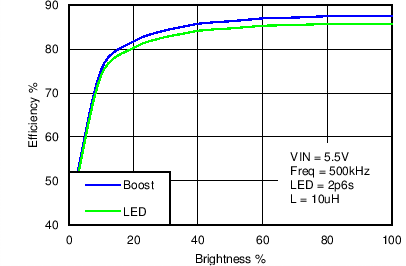VIN = 5.5 V 2p6s L = 10 µH Frequency = 500 kHz
Figure 10. Boost and LED EfficiencyVIN = 2.7 V 1p10s L = 22 µH Frequency = 500 kHz
Figure 12. Boost and LED Efficiency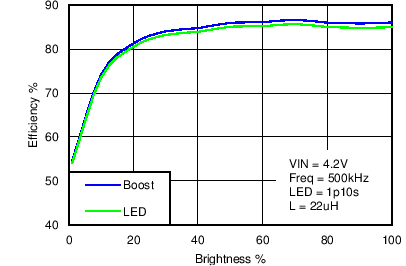VIN = 4.2 V 1p10s L = 22 µH Frequency = 500 kHz
Figure 14. Boost and LED Efficiency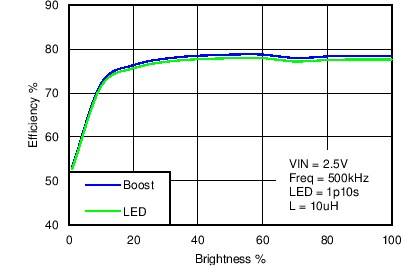VIN = 2.5 V 1p10s L = 10 µH Frequency = 500 kHz
Figure 16. Boost and LED Efficiency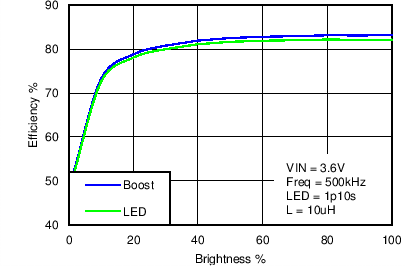VIN = 3.6 V 1p10s L = 10 µH Frequency = 500 kHz
Figure 18. Boost and LED Efficiency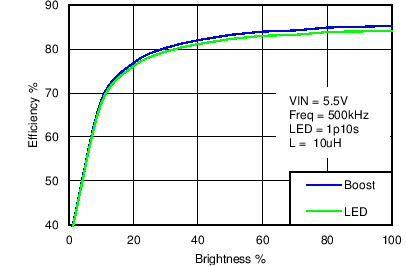VIN = 5.5 V 1p10s L = 10 µH Frequency = 500 kHz
Figure 20. Boost and LED Efficiency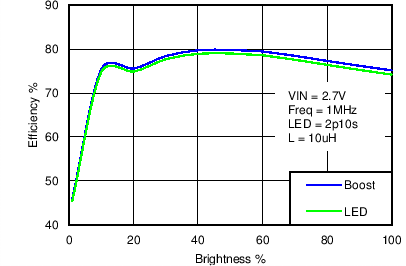VIN = 2.7 V 2p10s L = 10 µH Frequency = 1 MHz
Figure 22. Boost and LED Efficiency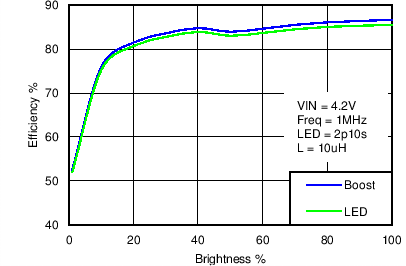VIN = 4.2 V 2p10s L = 10 µH Frequency = 1 MHz
Figure 24. Boost and LED Efficiency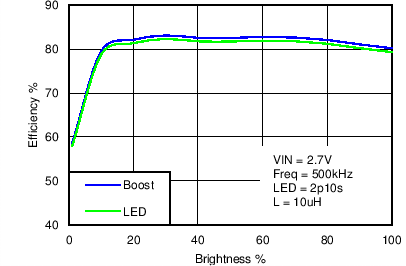VIN = 2.7 V 2p10s L = 10 µH Frequency = 500 kHz
Figure 26. Boost and LED Efficiency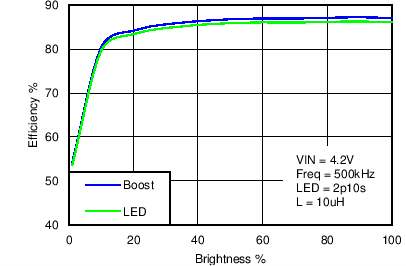VIN = 4.2 V 2p10s L = 10 µH Frequency = 500 kHz
Figure 28. Boost and LED EfficiencyILED Full Scale = 28.5 mA LED1 and 2 on DACA Frequency = 500 kHz 2p6s L = 10 µH
Figure 30. IIN Across VIN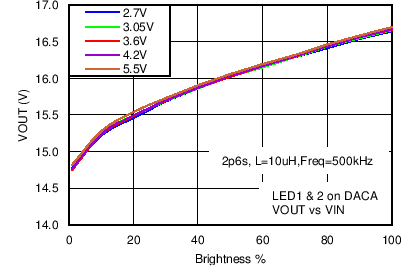ILED Full Scale = 28.5 mA LED1 and 2 on DACA Frequency = 500 kHz 2p6s L = 10 µH
Figure 32. VOUT Across VIN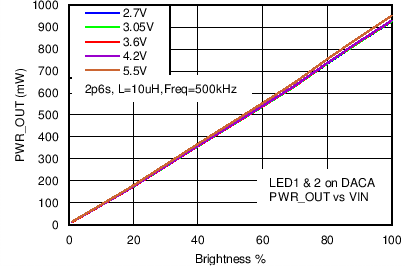ILED Full Scale = 28.5 mA LED1 and 2 on DACA Frequency = 500 kHz 2p6s L = 10 µH
Figure 34. PWR_OUT Across VINILED Full Scale = 28.5 mA LED1 and 2 on DACA Frequency = 500 kHz 2p6s L = 10 µH
Figure 36. I_INDUCTOR Across VIN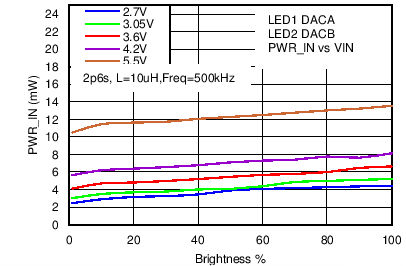ILED Full Scale = 28.5 mA LED1 on DACA 2p6s Frequency = 500 kHz LED2 on DACB L = 10 µH
Figure 38. PWR_IN Across VIN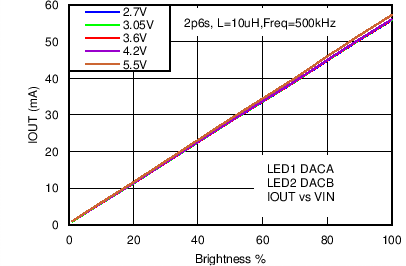ILED Full Scale = 28.5 mA LED1 on DACA 2p6s Frequency = 500 kHz LED2 on DACB L = 10 µH
Figure 40. IOUT Across VIN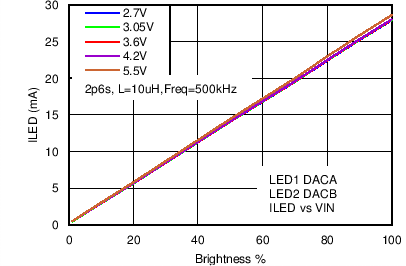ILED Full Scale = 28.5 mA LED1 on DACA 2p6s Frequency = 500 kHz LED2 on DACB L = 10 µH
Figure 42. ILED Across VIN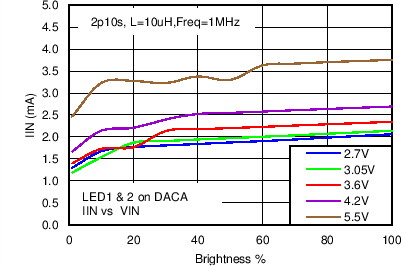ILED Full Scale = 28.5 mA LED1 and LED2 on DACA Frequency = 1 MHz 2p10s L = 10 µH
Figure 44. IIN Across VIN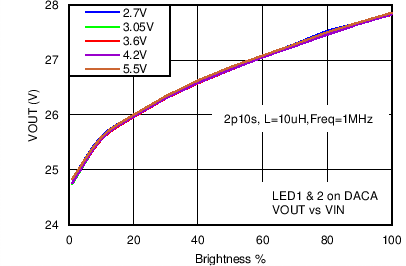ILED Full Scale = 28.5 mA LED1 and LED2 on DACA Frequency = 1 MHz 2p10s L = 10 µH
Figure 46. VOUT Across VINILED Full Scale = 28.5 mA LED1 and LED2 on DACA Frequency = 1 MHz 2p10s L = 10 µH
Figure 48. PWR_OUT Across VINILED Full Scale = 28.5 mA LED1 and LED2 on DACA Frequency = 1 MHz 2p10s L = 10 µH
Figure 50. I_INDUCTOR Across VINILED Full Scale = 28.5 mA LED1 on DACA 2p10s Frequency = 1 MHz LED2 on DACB L = 10 µH
Figure 52. PWR_IN Across VIN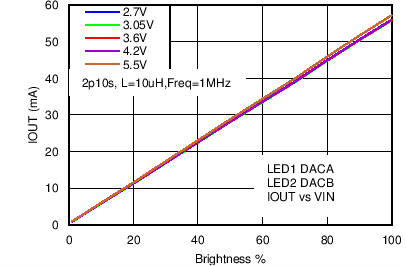ILED Full Scale = 28.5 mA LED1 on DACA 2p10s Frequency = 1 MHz LED2 on DACB L = 10 µH
Figure 54. IOUT Across VIN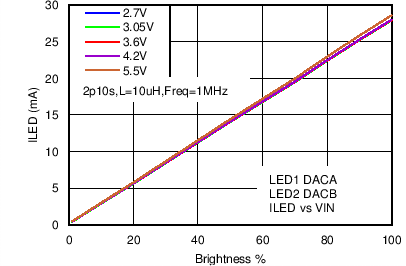ILED Full Scale = 28.5 mA LED1 on DACA 2p10s Frequency = 1 MHz LED2 on DACB L = 10 µH
Figure 56. ILED Across VINILED Full Scale = 28.5 mA LED1 and LED2 on DACA Frequency = 500 kHz 2p6s L = 22 µH
Figure 58. IIN Across VIN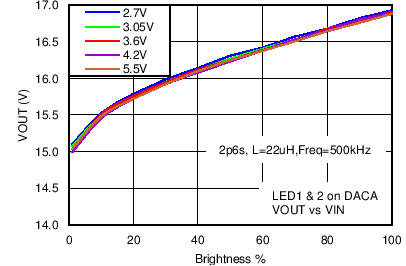ILED Full Scale = 28.5 mA LED1 and LED2 on DACA Frequency = 500 kHz 2p6s L = 22 µH
Figure 60. VOUT Across VIN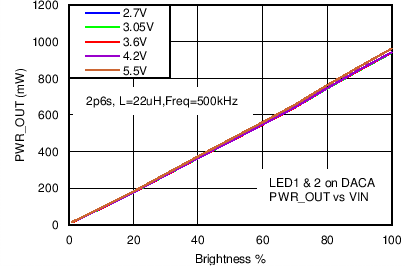ILED Full Scale = 28.5 mA LED1 and LED2 on DACA Frequency = 500 kHz 2p6s L = 22 µH
Figure 62. PWR_IOUT Across VINILED Full Scale = 28.5 mA LED1 and LED2 on DACA Frequency = 500 kHz 2p6s L = 22 µH
Figure 64. I_INDUCTOR Across VIN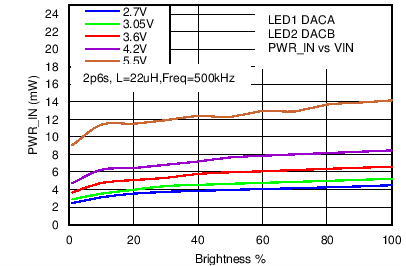ILED Full Scale = 28.5 mA LED1 on DACA 2p6s Frequency = 500 kHz LED2 on DACB L = 22 µH
Figure 66. PWR_IIN Across VIN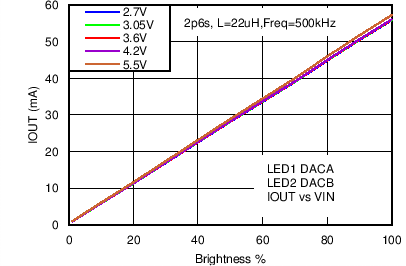ILED Full Scale = 28.5 mA LED1 on DACA 2p6s Frequency = 500 kHz LED2 on DACB L = 22 µH
Figure 68. IOUT Across VIN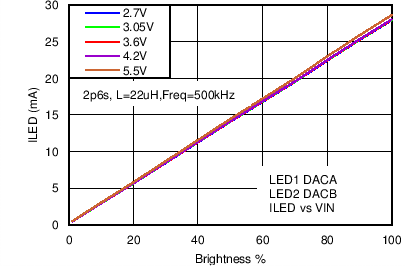ILED Full Scale = 28.5 mA LED1 on DACA 2p6s Frequency = 500 kHz LED2 on DACB L = 22 µH
Figure 70. ILED Across VIN